First an example of Thread is given in it's simplest form.  When used in this
simple form Thread takes an expression with arguments that are all lists of
equal length.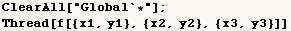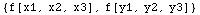In the next cell we see Thread automatically adapts if one or more elements
aren't lists.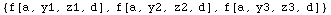The next three examples have frequent applications.  They have the same form
an example above but it may not look that way because infix operators
(→, ==) are used.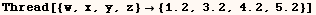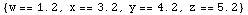As indicated above Thread works over lists by default.  In the next example
some of the expressions at level 1 of (lst1) aren't lists.  In a case such as
this the expressions that aren't lists are copied.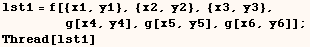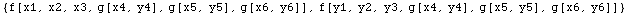Thread will work over a different head if one is provided as a second
argument.  The next line threads the same expression as the last one, but
last example expressions that didn't have the head (g) were copied.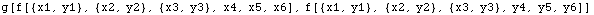In the next line Thread is provided a second and third argument.  The third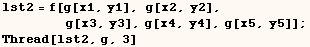In the next line Thread is given a third argument which says to only thread
over arguments 2 through 4.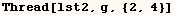Finally Thread is given (-3) as a third argument.  This means to only thread
over the last three arguments.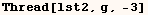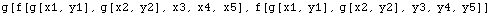listable functions across the head Equal.  The program is posted in
MathSource under Enhancements, Algebraic, 0202-204.  The code for this
enhancement is shown in the next cell.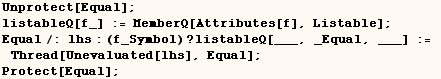The program above allows one to 'add' two equations in the next cell.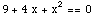Created by Mathematica  (May 16, 2004)

Back to Ted’s Tricks index page